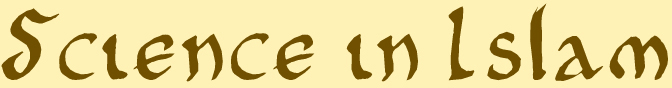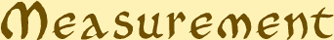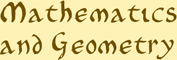## Mathematics & MeasurementMeasurement

Mathematics enabled Muslim scientists to make extraordinarily precise measurements and calculations of great significance.

At the beginning of the 9th century, under the Caliph al-Ma’mun, the first accurate calculation of the Earth’s circumference at the equator was made by determining the precise distance between two degrees of longitude. The measurements were made using instruments such as the quadrant or astrolabe, rods and stretched line in the plains of northern Iraq. The final measurement was 40,000km, a remarkably accurate figure.Mathematics & Geometry

Building on the work of their predecessors, Muslim scholars made a unique contribution to areas such as arithmetic, algebra, geometry and trigonometry.

Algebra (from the Arabic word “al-jabr” meaning “restoring balance”) was largely developed by the Persian mathematician Al-Khwarizmi (770-840) who was particularly interested in the practical applications of arithmetic in trade, law and engineering. The development of trigonometry was particularly important to astronomers and for its practical applications in surveying and construction.

Mathematics conveyed a symbolic significance in which numbers corresponded to a metaphysical realm of reality. This was clearly expressed in elaborate geometric designs used in architecture. These designs were a powerful representation of the divine unity.

Related objects:48472: Horizontal Pin-Gnomon Dial & Qibla Indicator, Persian, 18th Century?35285: Persian Quadrant, 1781/2